# How to Calculate and Solve for Effective Heat of Fusion | Solidification of Metals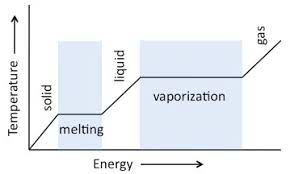The image above represents effective heat of fusion.

To compute for effective heat of fusion, three essential parameters are needed and these parameters are Latent Heat of Fusion (Hf), Heat Capacity at Constant Pressure (cp) and Change in Temperature (ΔT).

The formula for calculating effective heat of fusion:

H = Hf + cpΔT

Where:

H = Effective Heat of Fusion
Hf = Latent Heat of Fusion
cp = Heat Capacity at Constant Pressure
ΔT = Change in Temperature

Let’s solve an example;
Find the effective heat of fusion when the latent heat of fusion is 12, the heat capacity at constant pressure is 14 and the change in temperature is 10.

This implies that;

Hf = Latent Heat of Fusion = 12
cp = Heat Capacity at Constant Pressure = 14
ΔT = Change in Temperature = 10

H = Hf + cpΔT
H = 12 + 14(10)
H = 12 + 140
H = 152

Therefore, the effective heat of fusion is 152 J/Kg.

Calculating the Latent Heat of Fusion when the Effective Heat of Fusion, the Heat Capacity at Constant Pressure and the Change in Temperature is Given.

Hf = H – cpΔT

Where:

Hf = Latent Heat of Fusion
H = Effective Heat of Fusion
cp = Heat Capacity at Constant Pressure
ΔT = Change in Temperature

Let’s solve an example;
Find the latent heat of fusion when the effective heat of fusion is 42, the heat capacity at constant pressure is 10 and the change in temperature is 2.

This implies that;

H = Effective Heat of Fusion = 42
cp = Heat Capacity at Constant Pressure = 10
ΔT = Change in Temperature = 2

Hf = H – cpΔT
Hf = 42 – (10)(2)
Hf = 42 – 20
Hf = 22

Therefore, the latent heat of fusion is 22.

Calculating the Heat Capacity at Constant Pressure when the Effective Heat of Fusion, the Latent Heat of Fusion and the Change in Temperature is Given.

cp = H – Hf / ΔT

Where:

cp = Heat Capacity at Constant Pressure
H = Effective Heat of Fusion
Hf = Latent Heat of Fusion
ΔT = Change in Temperature

Let’s solve an example;
Find the heat capacity at constant pressure when the effective heat of fusion is 20, the latent heat of fusion is 8 and the change in temperature is 4.

This implies that;

H = Effective Heat of Fusion = 20
Hf = Latent Heat of Fusion = 8
ΔT = Change in Temperature = 4

cp = H – Hf / ΔT
cp = 20 – 8 / 4
cp = 12 / 4
cp = 3

Therefore, the heat capacity at constant temperature is 3.

Calculating the Change in Temperature when the Effective Heat of Fusion, the Latent Heat of Fusion and the Heat Capacity at Constant Pressure is Given.

ΔT = H – Hf / cp

Where:

ΔT = Change in Temperature
H = Effective Heat of Fusion
Hf = Latent Heat of Fusion
cp = Heat Capacity at Constant Pressure

Let’s solve an example;
Find the change in temperature when the effective heat of fusion is 30, the latent heat of fusion is 6 and the heat capacity at constant pressure is 8.

This implies that;

H = Effective Heat of Fusion = 30
Hf = Latent Heat of Fusion = 6
cp = Heat Capacity at Constant Pressure = 8

ΔT = H – Hf / cp
ΔT = 30 – 6 / 8
ΔT = 24 / 8
ΔT = 3

Therefore, the change in temperature is 3.

Nickzom Calculator – The Calculator Encyclopedia is capable of calculating the effective heat of fusion.

To get the answer and workings of the effective heat of fusion using the Nickzom Calculator – The Calculator Encyclopedia. First, you need to obtain the app.

You can get this app via any of these means:

To get access to the professional version via web, you need to register and subscribe for NGN 2,000 per annum to have utter access to all functionalities.
You can also try the demo version via https://www.nickzom.org/calculator

Apple (Paid) – https://itunes.apple.com/us/app/nickzom-calculator/id1331162702?mt=8
Once, you have obtained the calculator encyclopedia app, proceed to the Calculator Map, then click on Materials and Metallurgical under Engineering.Now, Click on Solidification of Metal under Materials and Metallurgical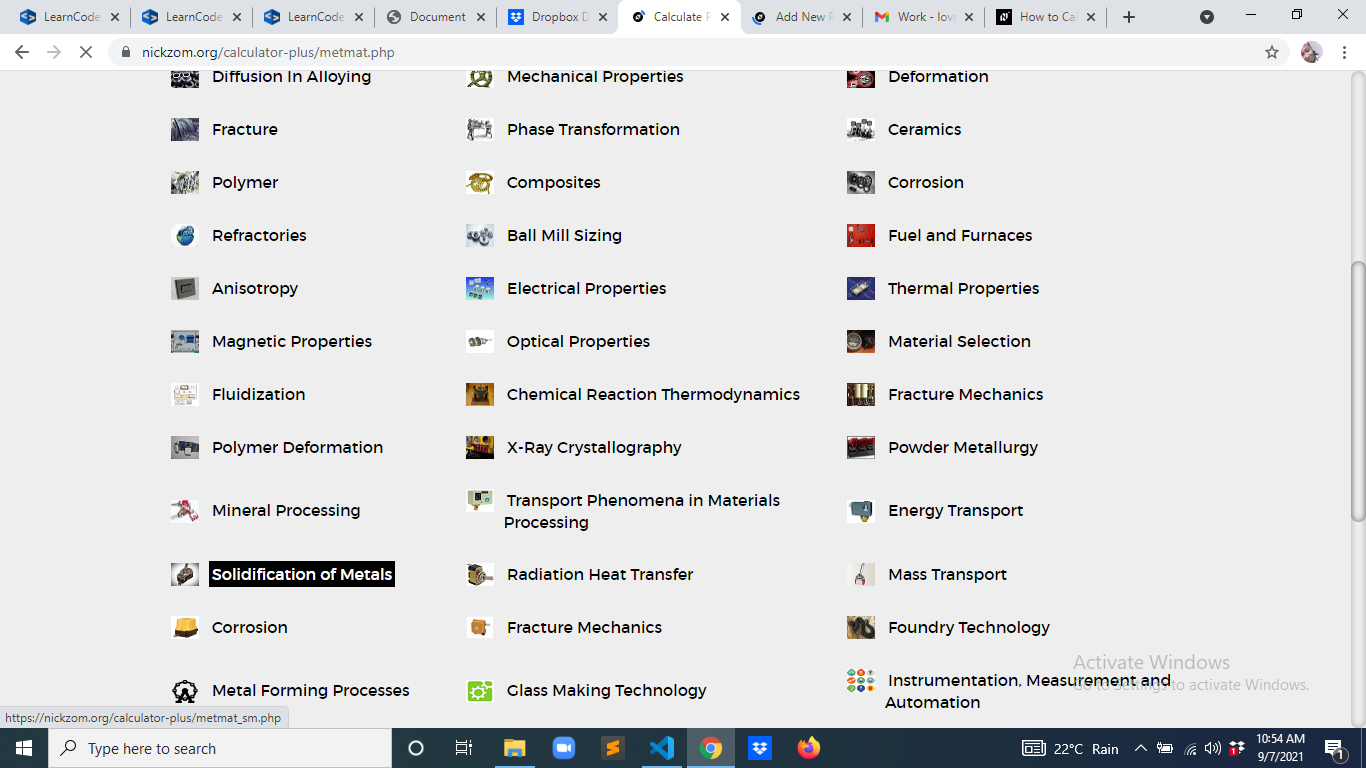Now, Click on Effective Heat of Fusion under Solidification of Metal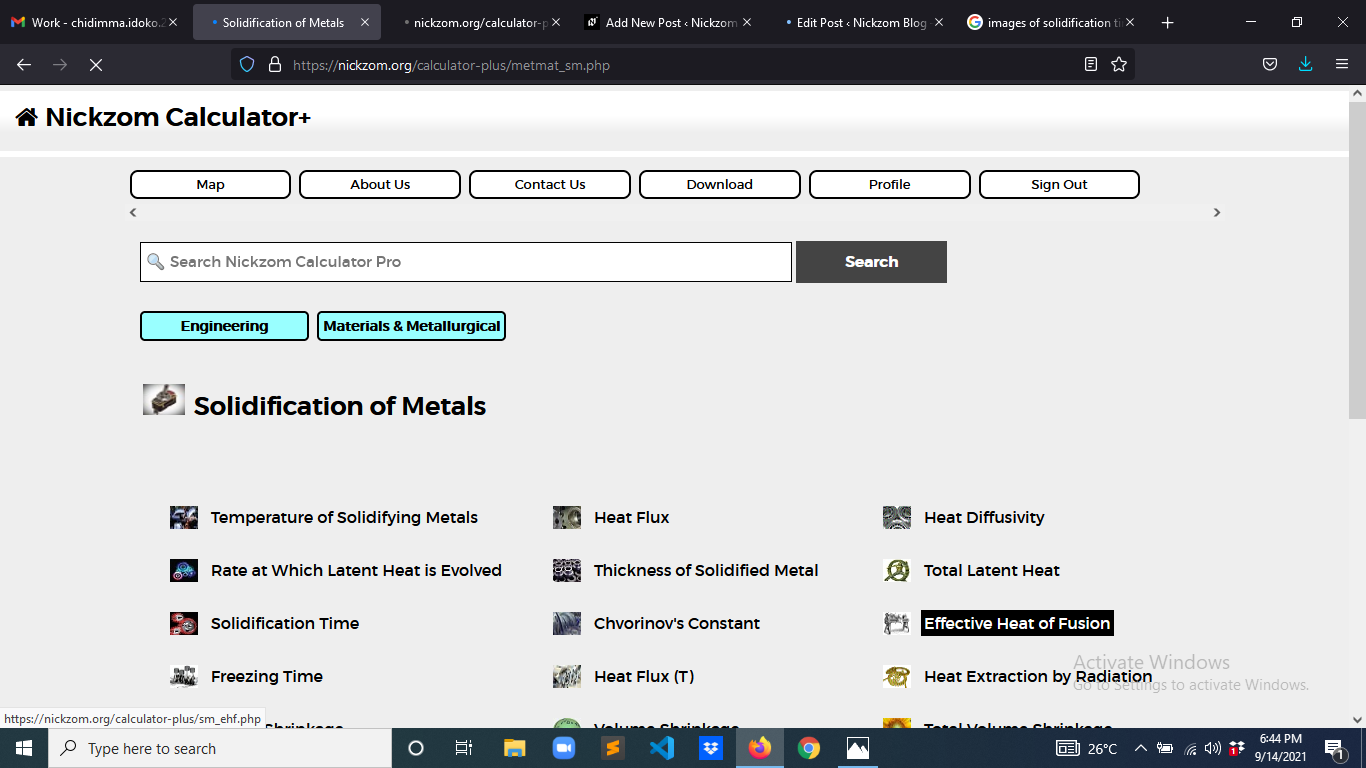The screenshot below displays the page or activity to enter your values, to get the answer for the effective heat of fusion according to the respective parameter which is the Latent Heat of Fusion (Hf), Heat Capacity at Constant Pressure (cp) and Change in Temperature (ΔT).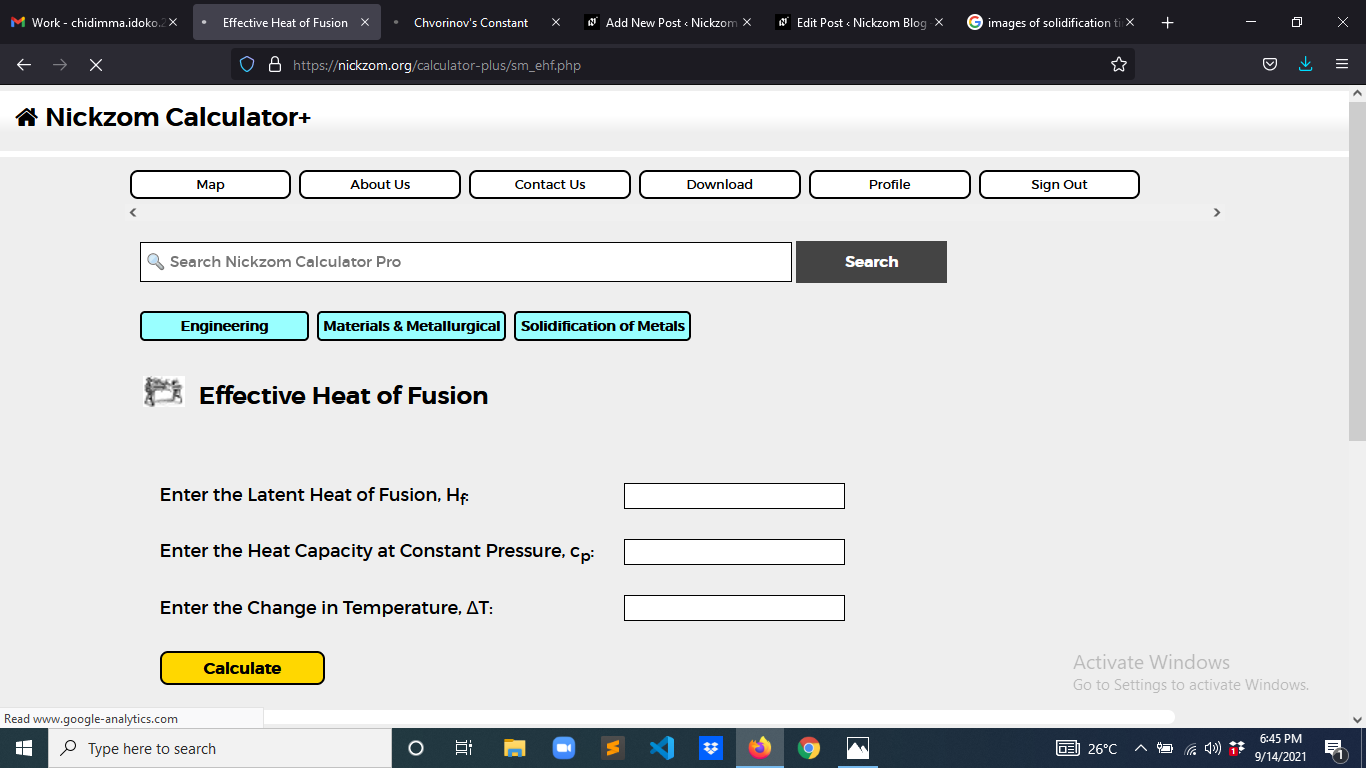Now, enter the values appropriately and accordingly for the parameters as required by the Latent Heat of Fusion (Hf) is 12, Heat Capacity at Constant Pressure (cp) is 14 and Change in Temperature (ΔT) is 10.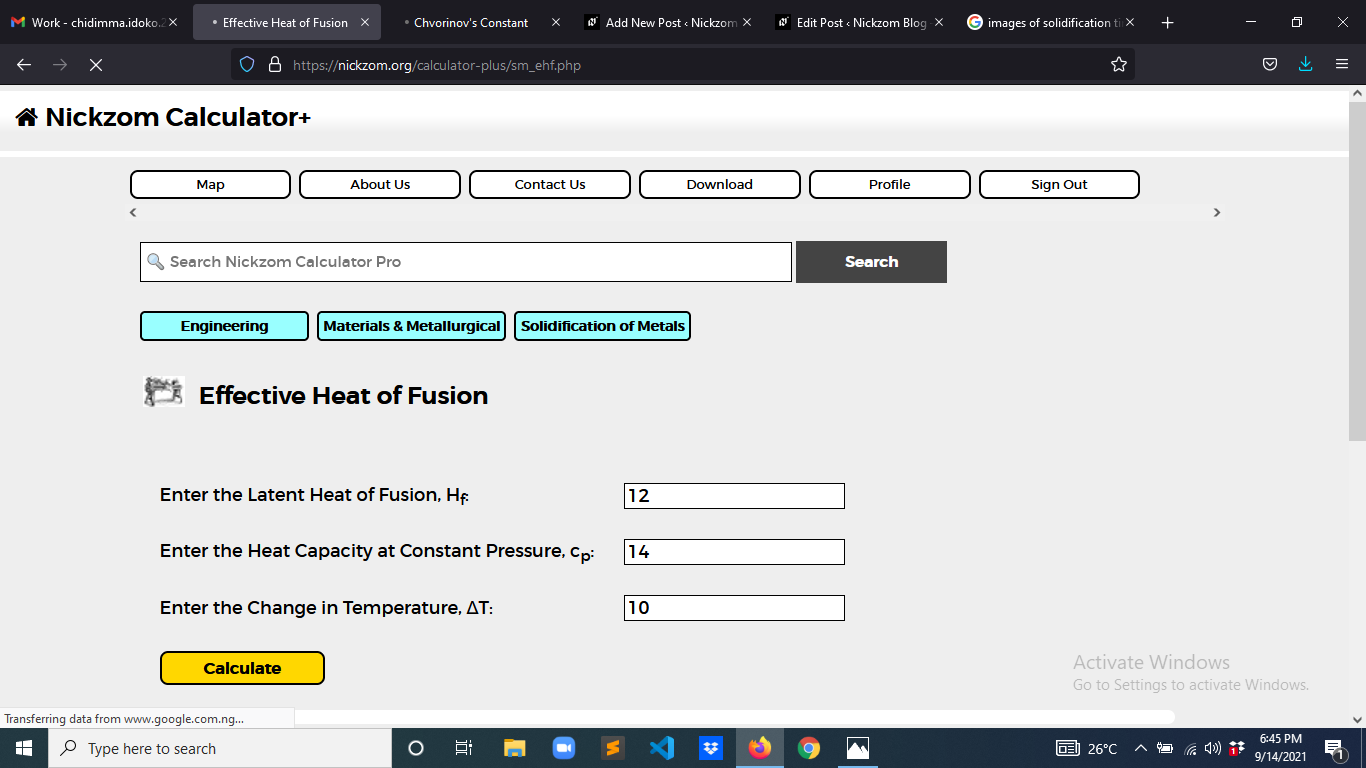Finally, Click on Calculate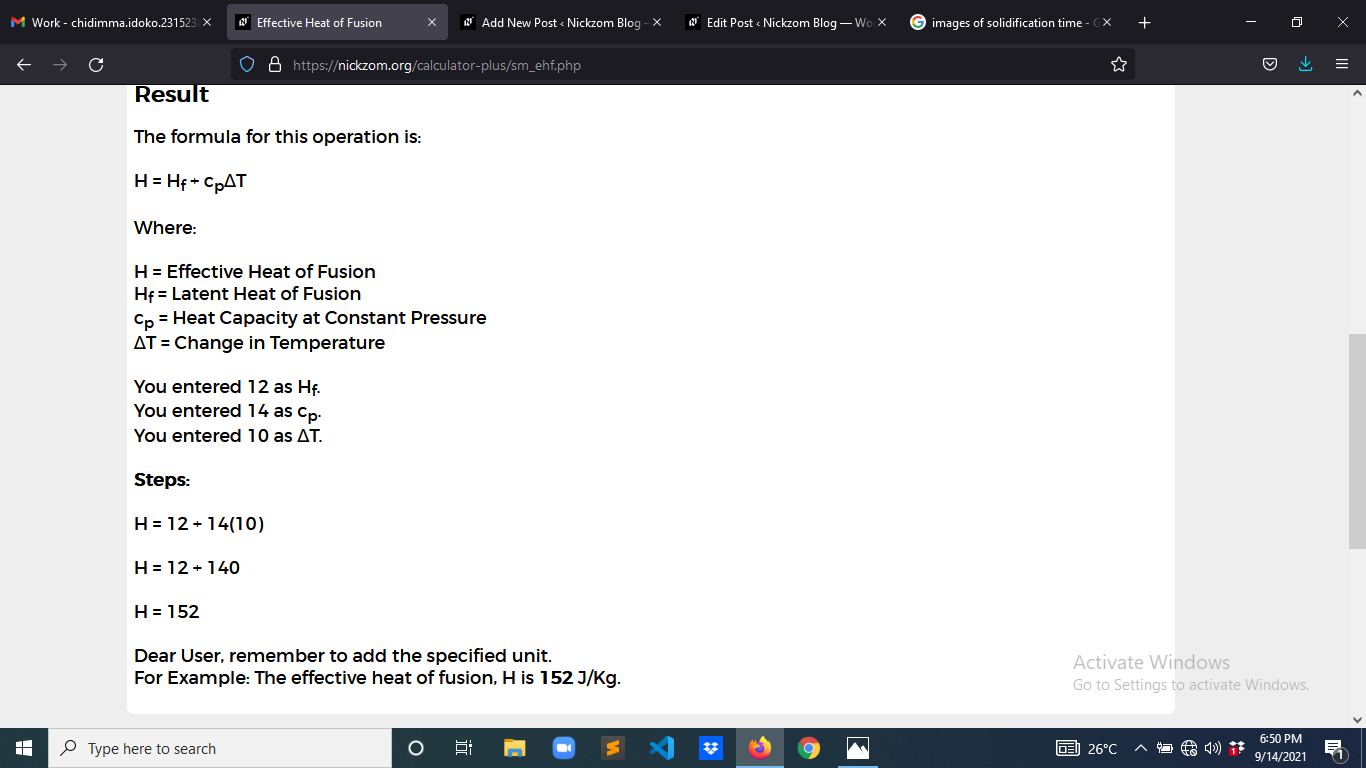As you can see from the screenshot above, Nickzom Calculator– The Calculator Encyclopedia solves for the effective heat of fusion and presents the formula, workings and steps too.